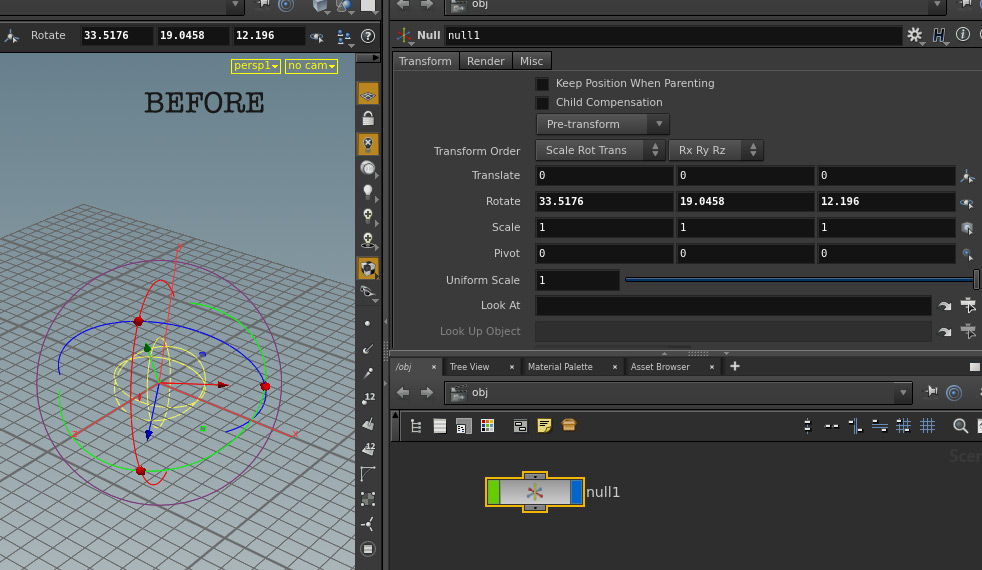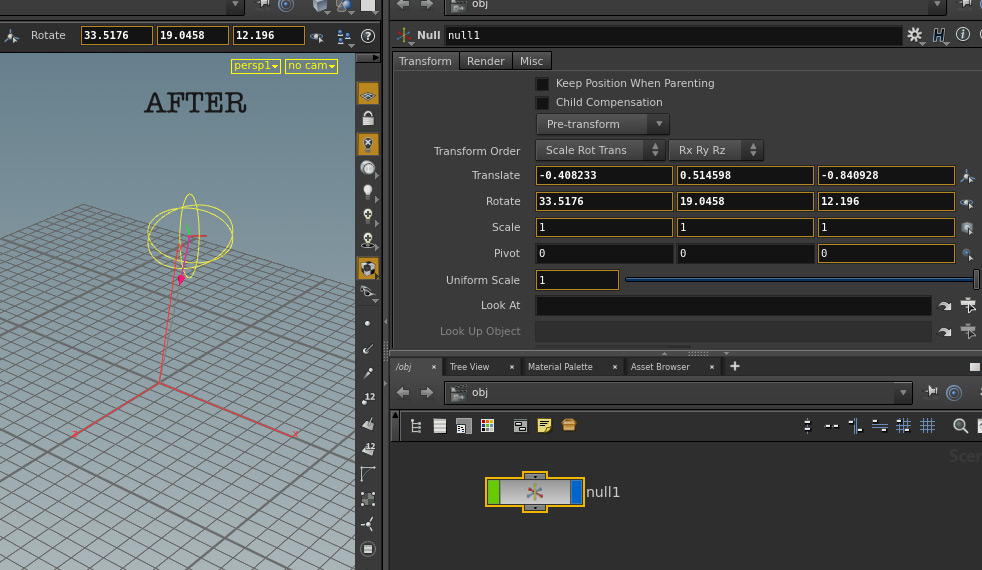## Recommended Posts

HI All,

I have a NULL that is hanging out in space.  Let's say it is randomly rotated as well.

I want to calculate a new world position for that NULL that is X units away from it's current position, projected along it's orientation axis. Kind of like a particle multiplied by it's velocity for the next frame position.

I can fetch the various portions of the object matrix but I don't know how to operate upon them to calculate the new position?

```n = hou.node("/obj/geo1")
if n != None:
distance_to_project = 2.0
mtx = n.worldTransform()
pos=mtx.extractTranslates()
rot=mtx.extractRotates()```

Edited by Atom

##### Share on other sites

You can construct a matrix that represents this x-axis transform and then multiply that matrix by the original transform.

```n = hou.node("/obj/geo1")
if n:
mtx = n.worldTransform()
translate_x = hou.hmath.buildTranslate((2,0,0))
mtx = translate_x * mtx
n.setWorldTransform(mtx)```

##### Share on other sites

Thanks for the code, that is close. But how do I factor in the distance moved and the orientation? Also it is not just along the x-axis.

The image shows my problem. In the viewport I can mouse along the z-axis to move the NULL, which controls the lip morph. However, if I observe the Translate area I can see that the Y and Z translation values are changing even though I have not dragged along the Y-axis at all. Houdini is calculating a new projected coordinate based upon my mouse move.

I need to project along the Z-axis at the current angle to produce a new location. The end goal is to link this projection to a slider on a python node to help form facial phonemes. The slider will provide a distance value to the final equation.

Edited by Atom

##### Share on other sites

I'm not exactly sure what you mean, but it sounds like your NULLs parent transform is not oriented the way you want.  I think all you need to do is clean your transform.

EDIT : Wait that's not what you mean is it?

What your looking for is basically something analogous to SDKs in Maya?

Edited by MrScienceOfficer

##### Share on other sites

Think of the NULL as a particle. How does a particle get it's new location from frame-to-frame? It starts in one location then moves to another along the distance of it's orientation. The distance would be considered it's velocity, right... I don't know the math to get from one location to another along an oriented distance.

The orientation would be the rotation of the NULL and the velocity would be a supplied float.

Edited by Atom

##### Share on other sites

I don't see anything wrong with MirrorSword's code snippet. If you have an arbitrary direction normalized unit vector v, then the math is just "v * distance". In MirrorSword's code snippet, you just use "v * distance" instead of (2,0,0).

##### Share on other sites

Ok so I am down to one missing line of code.

Can anyone fill in the missing line of code below?

```n = hou.node("/obj/geo1")
if n:
dist = node.parm("distance").eval()
mtx = n.worldTransform()
missing_line_of_code = dist * "arbitrary direction normalized unit vector v"
translate_all_three_axis = hou.hmath.buildTranslate(missing_line_of_code)
mtx = translate_all_three_axis * mtx
n.setWorldTransform(mtx)
```

##### Share on other sites

I'm not 100% sure I understand what you are looking for. But here are some ideas.

This will move it relative to object space with a distance and normalized direction.

```n = hou.node("/obj/geo1")
if n:
mtx = n.worldTransform()
distance = 2
direction = hou.Vector3(1,0,0).normalized()
vector = direction * distance
translate_matrix = hou.hmath.buildTranslate(vector)
mtx = translate_matrix * mtx
n.setWorldTransform(mtx)```

To summarize this, you get a distance and a normalized direction, and then multiply them together to make your offset vector. Then you use that vector to build a matrix that translates along that vector. Then you multiply that matrix with your objects matrix. This has the effect of moving the object relative to object space.

Alternatively if you just want a velocity vector that is transformed by your objects matrix you can do it with the following:

```n = hou.node("/obj/geo1")
if n:
v1 = hou.Vector4(2,0,0,0)
mtx = n.worldTransform()
v2 = v1 * mtx
v2 = hou.Vector3(v2)
print v2```

(v1 needs to be a vector4 with a zero as the 4th component so that it is transformed as a velocity and not as a position, as explained here)

•1

##### Share on other sites

You could parent another null to your null and use the parent null for rotation and the child null for the Z-axis movement.

Alternatively you could change your transform order so translates are calculated first, which should work as expected if you only intend on moving that control with a parm.

Using python for each control on your characters face would likely hinder both performance and flexibility on your rig.

##### Share on other sites

That is very close, but the direction needs to come from the current rotation value.

Here is the NULL before I use the mouse and drag it along the Z-axis.Here is NULL after I drag it along the Z-axis in the viewport.Notice that the XY and Z values in the Translate field have changed. This is because I am not just moving it along the Z coordinate. The new location is being projected along the Z axis based upon the current rotation.

So I guess I am still back to needing one line of missing code.

How do I convert the current rotation into a vector?

```node = hou.pwd()

n = hou.node("/obj/null1")
if n:
mtx = n.worldTransform()
distance = node.parm("dist").eval()
missing_rotation_to_direction_code = something
direction = missing_rotation_to_direction_code.normalized()
vector = direction * distance
translate_matrix = hou.hmath.buildTranslate(vector)
mtx = translate_matrix * mtx
n.setWorldTransform(mtx)```

@MrScienceOfficer: Modifying the rig by adding more parts to it is not really a solution for this task. I will be processing a lot of rigs and the code needs to work with the FBX imported rig as is. As far as performance, that is at the bottom of the list. I mean what rig runs at realtime in the viewport anyway? You always have to render to see the results. And to quote Jeff Wagner, the voice of SideFX, "First get it to work, then optimize." I am still at the try to get it to work stage of the process.

##### Share on other sites

I believe my code actually does what you want. Note the order of the matrix multiplication. "translation_matrix * mtx" constructs a matrix that will first transform the object with the translate_matrix and then transform the object with the original object matrix (mtx). So in my example it should move the object along the local x-axis, not the world x-axis. If the matrix multiplication was reversed ("mtx * translate_matrix") then the code would behave incorrectly and translate in world space instead of object space.

##### Share on other sites

I agree it is very close, I am just trying to integrate the rotation portion into the solution.

Here is my version of your modified code almost working.I am guessing at how to construct the rotation vector. If I supply angles or radians it still does not project along the correct vector. Is that the correct way to create the direction?

```import math
node = hou.pwd()

n = hou.node("/obj/null1")
if n:
#mtx = n.worldTransform()
mtx = hou.hmath.buildTranslate(hou.Vector3(0,0,0))
distance = node.parm("dist").eval()
else:
rx = n.parm("rx").eval()
ry = n.parm("ry").eval()
rz = n.parm("rz").eval()

v = hou.Vector3(rx, ry, rz)
direction = v.normalized()
vector = direction * distance
translate_matrix = hou.hmath.buildTranslate(vector)
mtx = translate_matrix * mtx
n.setWorldTransform(mtx)
```

ap_project_along_rotation.hiplc

##### Share on other sites

So you don't need to do anything specific with the rotations, the matrix math takes care of that. I think I updated your example to do what you want, but I had to add an extra null so I would have an original position to reference. (Hip file Attached)

```import math
node = hou.pwd()

n1 = hou.node("/obj/null1")
n2 = hou.node("/obj/null2")
if n1 and n2:
mtx = n1.worldTransform()
distance = node.parm("dist").eval()
v = hou.Vector3(0,0,1)
direction = v.normalized()
vector = direction * distance
translate_matrix = hou.hmath.buildTranslate(vector)
mtx = translate_matrix * mtx
n2.setWorldTransform(mtx)```

Also I'm not sure what you were trying to do here:

```    v = hou.Vector3(rx, ry, rz)
direction = v.normalized()
vector = direction * distance
translate_matrix = hou.hmath.buildTranslate(vector)```

but it seems like you are making a mistake with how you treat rotations. You can't convert a rotation into a vector by just plugging the x,y,z rotations into the x,y,z components of a vector as they represent completely different things. If you want to create a vector that is relative to a rotation, you should do it by first creating the vector in local space then rotating it by a matrix.

Like so:

```v1 = hou.Vector4(2,0,0,0)
rx = 30
ry = 30
rz = 30
mtx = hou.hmath.buildRotate((rx,ry,rz),"xyz")
v2 = v1 * mtx
v2 = hou.Vector3(v2)
print v2```

ap_project_along_rotation_v2.hiplc

•1

##### Share on other sites

Yep, that is it!

Thanks for working through this with me.•1

## Create an account

Register a new account